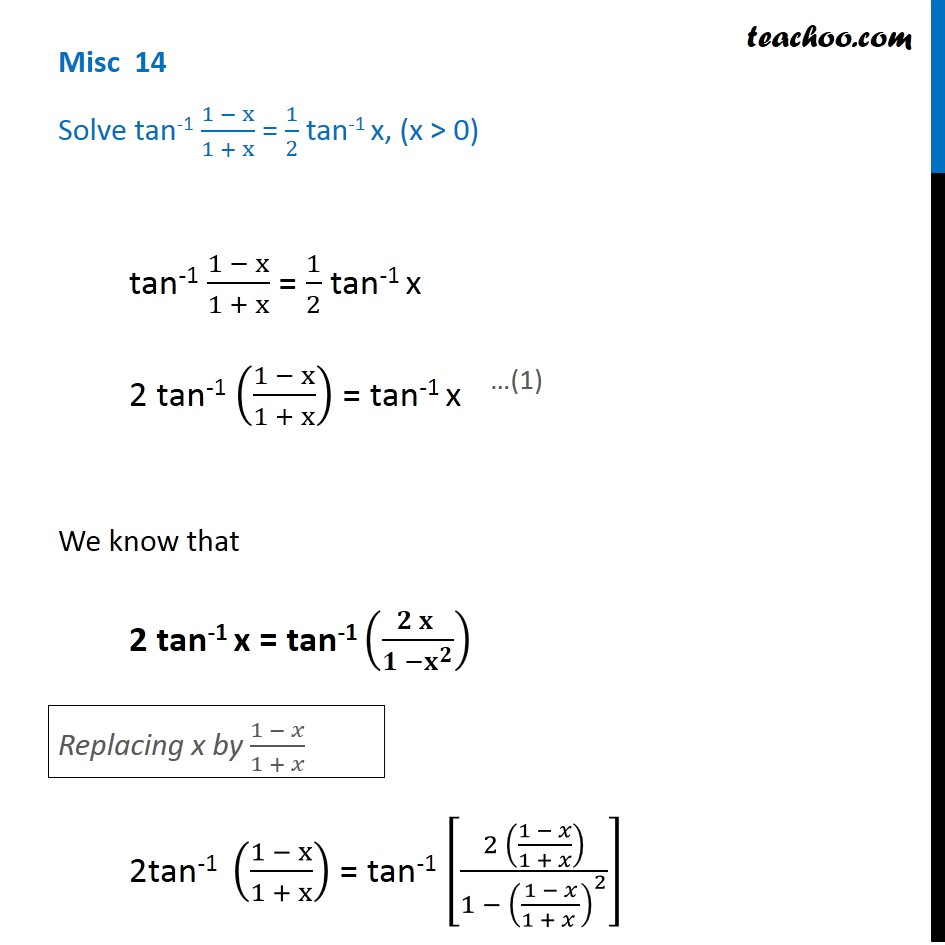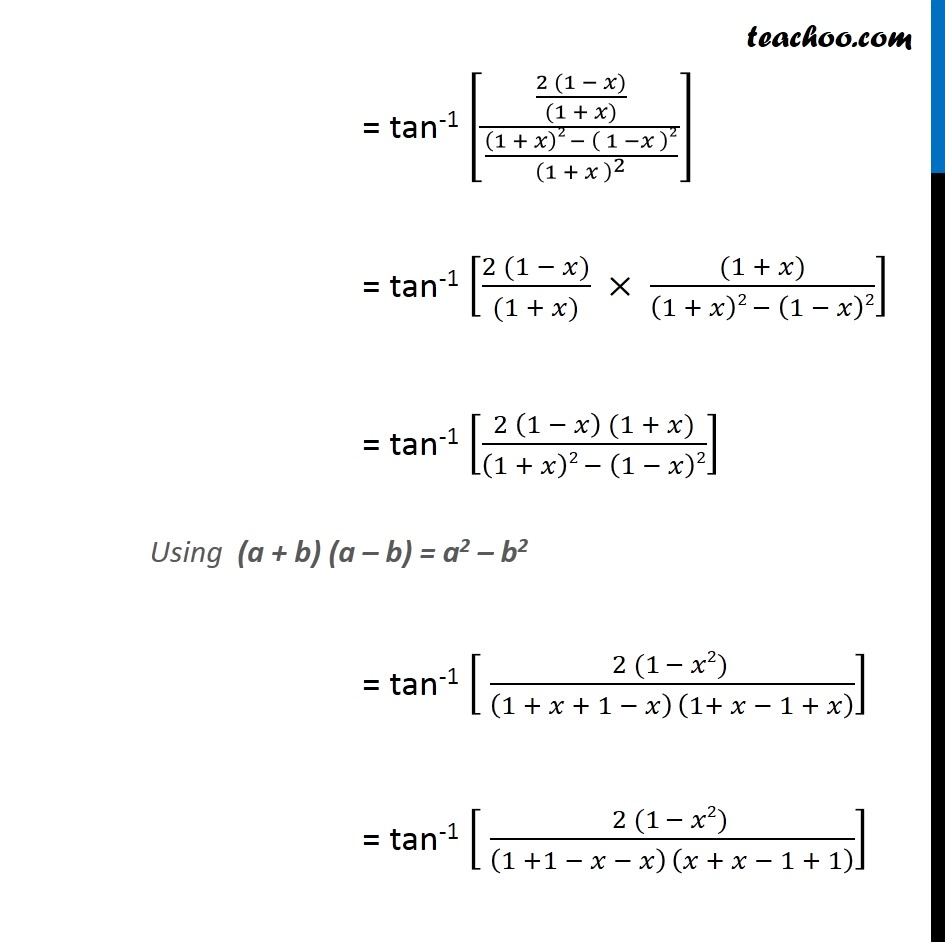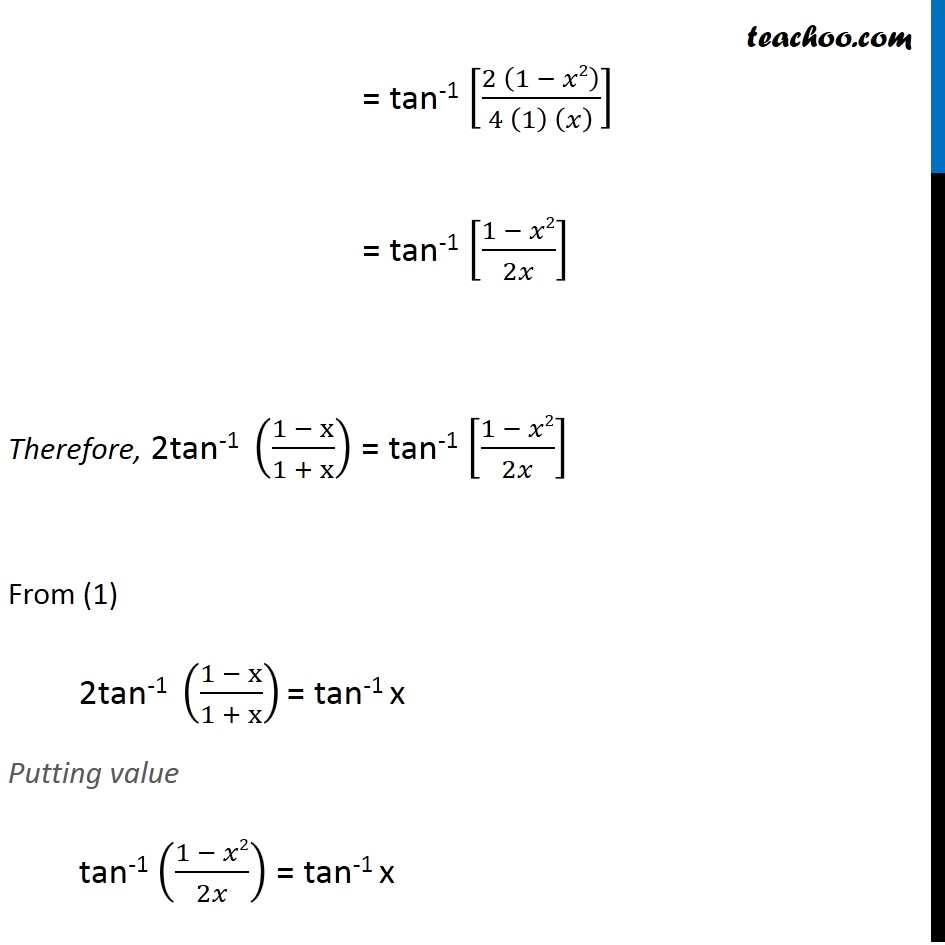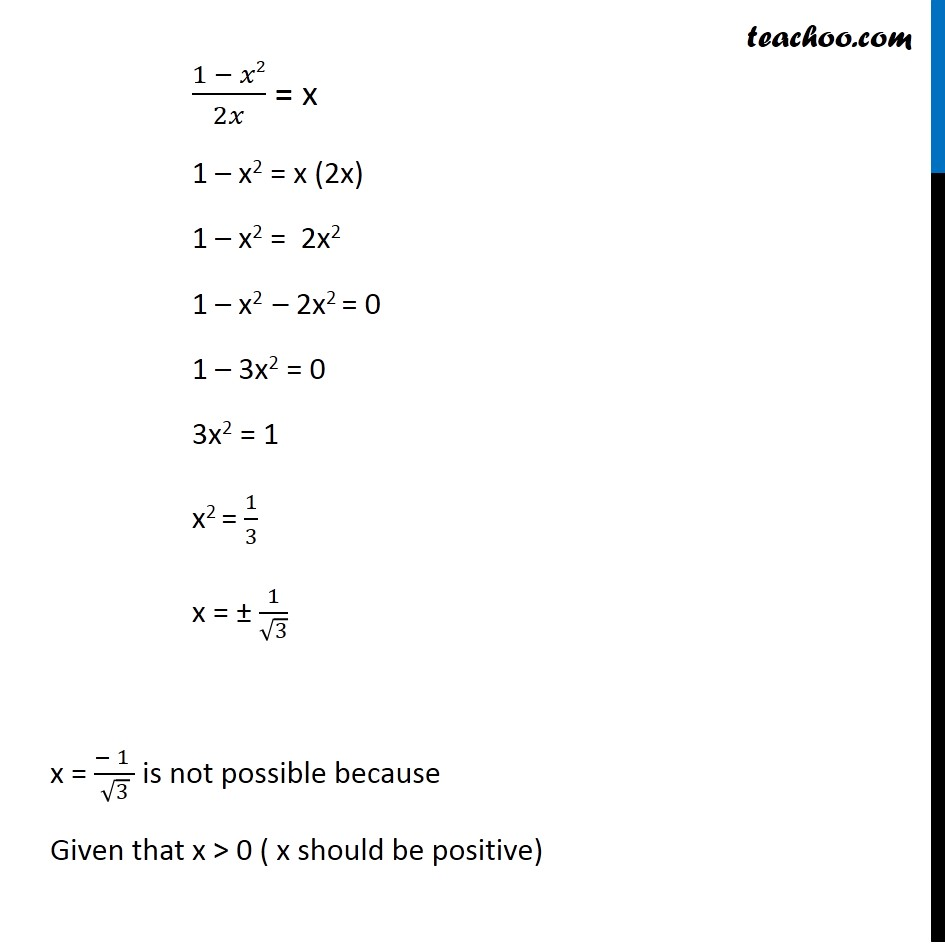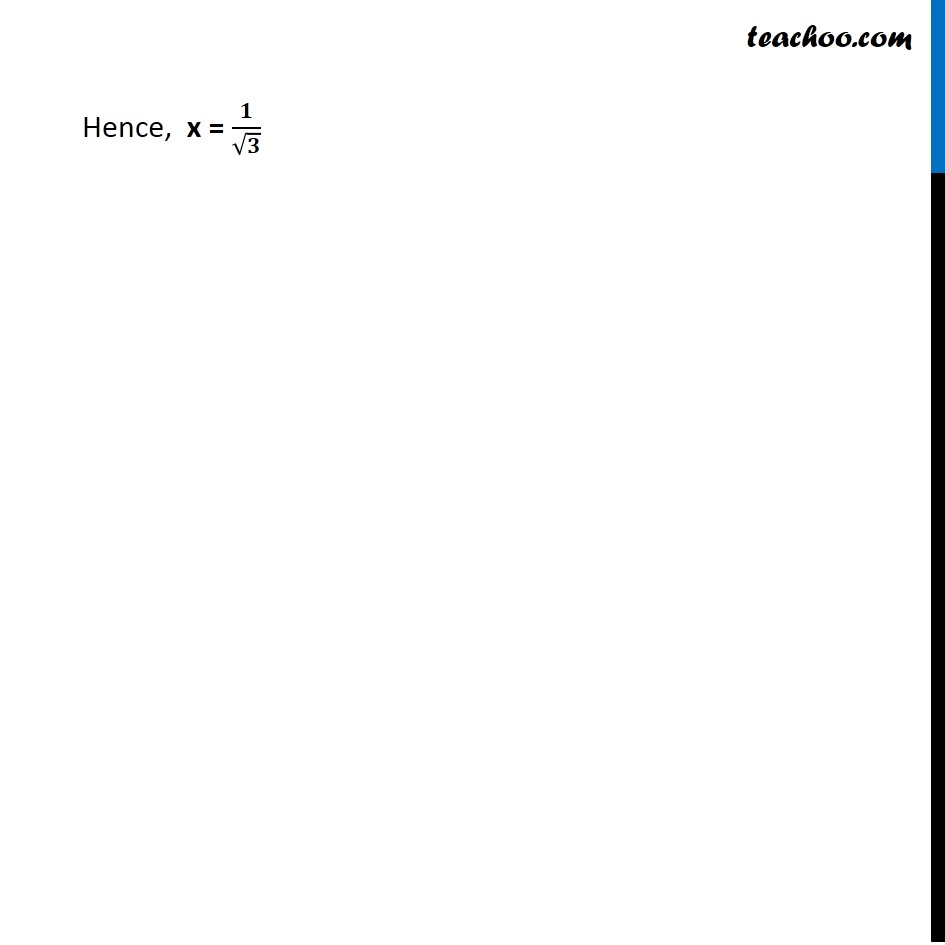Subscribe to our Youtube Channel - https://you.tube/teachoo

1. Chapter 2 Class 12 Inverse Trigonometric Functions
2. Serial order wise
3. Miscellaneous

Transcript

Misc 14 Solve tan-1 (1 − x)/(1 + x) = 1/2 tan-1 x, (x > 0) tan-1 (1 − x)/(1 + x) = 1/2 tan-1 x 2 tan-1 ((1 − x)/(1 + x)) = tan-1 x We know that 2 tan-1 x = tan-1 ((𝟐 𝐱 )/(𝟏 −𝐱^𝟐 )) 2tan-1 ((1 − x)/(1 + x)) = tan-1 [(2 ((1 − 𝑥)/(1 + 𝑥)))/(1 − ((1 − 𝑥)/(1 + 𝑥 ))^2 )] Replacing x by (1 − 𝑥)/(1 + 𝑥) = tan-1 [((2 (1 − 𝑥))/((1 + 𝑥)))/(((1 + 𝑥)2 − ( 1 −𝑥 )2)/(1 + 𝑥 )^2 )] = tan-1 [(2 (1 − 𝑥))/((1 + 𝑥)) × ((1 + 𝑥))/((1 + 𝑥)2 − (1 − 𝑥)2)] = tan-1 [(2 (1 − 𝑥) (1 + 𝑥))/((1 + 𝑥)2 − (1 − 𝑥)2)] Using (a + b) (a – b) = a2 – b2 = tan-1 [ (2 (1 − 𝑥2) )/((1 + 𝑥 + 1 − 𝑥) (1+ 𝑥 − 1 + 𝑥) )] = tan-1 [ (2 (1 − 𝑥2) )/((1 +1 − 𝑥 − 𝑥) (𝑥 + 𝑥 − 1 + 1) )] = tan-1 [(2 (1 − 𝑥2))/(4 (1) (𝑥) )] = tan-1 [(1 − 𝑥2)/2𝑥] Therefore, 2tan-1 ((1 − x)/(1 + x)) = tan-1 [(1 − 𝑥2)/2𝑥] From (1) 2tan-1 ((1 − x)/(1 + x)) = tan-1 x Putting value tan-1 ((1 − 𝑥2)/2𝑥) = tan-1 x (1 − 𝑥2)/2𝑥 = x 1 – x2 = x (2x) 1 – x2 = 2x2 1 – x2 – 2x2 = 0 1 – 3x2 = 0 3x2 = 1 x2 = 1/3 x = ± 1/√3 x = (− 1 )/√3 is not possible because Given that x > 0 ( x should be positive) Hence, x = ( 𝟏 )/√𝟑

Miscellaneous